/35
1

Best of Luck1 / 35

1) Which symbol will be on the face opposite to the face with symbol * ?2 / 35

2) Find out the alternative figure which contains figure (X) as its part.3 / 35

3) Two positions of a cube with its surfaces numbered are shown below. When surface 4 touches the bottom, what surface will be on the top?4 / 35

4) Choose a figure which would most closely resemble the unfolded form of Figure (Z).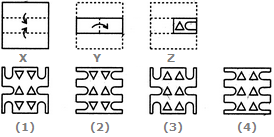5 / 35

5) Identify the figure that completes the pattern.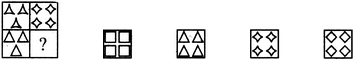6 / 35

6) Find out the alternative figure which contains figure (X) as its part.7 / 35

7) Choose a figure which would most closely resemble the unfolded form of Figure (Z).8 / 35

8) Identify the figure that completes the pattern.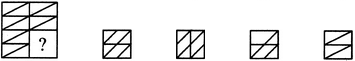9 / 35

9) Here two positions of dice are shown. If there are two dots in the bottom, then how many dots will be on the top?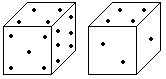10 / 35

10) Choose a figure which would most closely resemble the unfolded form of Figure (Z).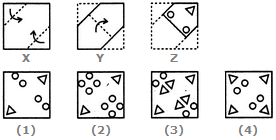11 / 35

11) Identify the figure that completes the pattern.12 / 35

12) Choose a figure which would most closely resemble the unfolded form of Figure (Z).13 / 35

13) Identify the figure that completes the pattern.14 / 35

14) Identify the figure that completes the pattern.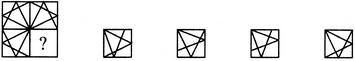15 / 35

15) Two positions of a dice are shown below. Which number will appear on the face opposite to the face with the number 5?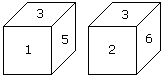16 / 35

16) How many points will be on the face opposite to in face which contains 2 points?<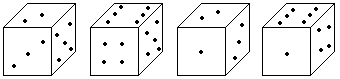17 / 35

17) Choose a figure which would most closely resemble the unfolded form of Figure (Z).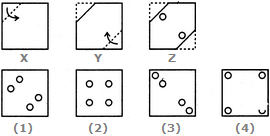18 / 35

18) Select a suitable figure from the four alternatives that would complete the figure matrix.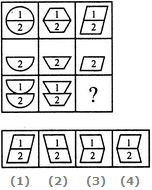19 / 35

19) Which number is on the face opposite to 6?20 / 35

20) Identify the figure that completes the pattern.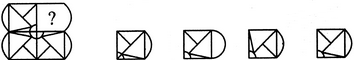21 / 35

21) Select a suitable figure from the four alternatives that would complete the figure matrix.22 / 35

22) Find out the alternative figure which contains figure (X) as its part.23 / 35

23) Two positions of dice are shown below. How many points will appear on the opposite to the face containing 5 points?24 / 35

24) Find out the alternative figure which contains figure (X) as its part.25 / 35

25) Identify the figure that completes the pattern.26 / 35

26) Select a suitable figure from the four alternatives that would complete the figure matrix.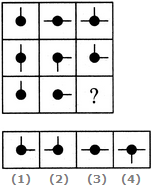27 / 35

27) Find out the alternative figure which contains figure (X) as its part.28 / 35

28) Which digit will appear on the face opposite to the face with the number 4?29 / 35

29) Identify the figure that completes the pattern.30 / 35

30) Two positions of dice are shown below. How many points will be on the top when 2 points are at the bottom?31 / 35

31) Select a suitable figure from the four alternatives that would complete the figure matrix.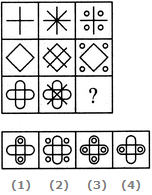32 / 35

32) Identify the figure that completes the pattern.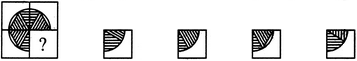33 / 35

33) Two positions of a dice are shown below. When number '1' is on the top. What number will be at the bottom?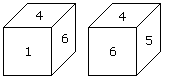34 / 35

34) Identify the figure that completes the pattern.35 / 35

35) Select a suitable figure from the four alternatives that would complete the figure matrix.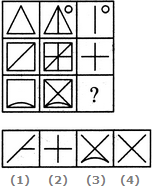0%